Function Repository Resource:

# TreeEditDistance

Calculate the edit distance between two Tree objects

Contributed by: Arnab Datta
 ResourceFunction["TreeEditDistance"][t1,t2] gives the edit distance between Tree objects t1 and t2.

## Details

The edit distance is the minimum number of node deletion, insertion and substitution operations needed to transform one tree into another.

## Examples

### Basic Examples (2)

Create two expression trees:

 In:=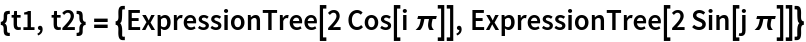Out=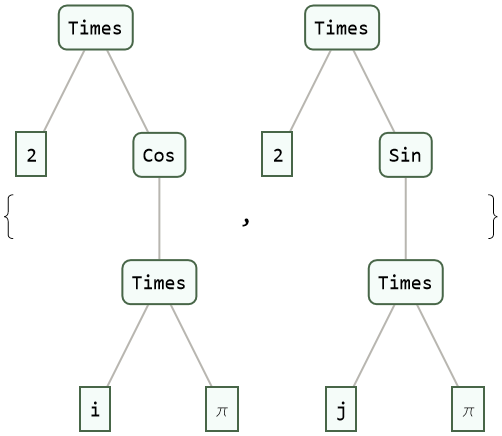Compute the edit distance between the two trees:

 In:=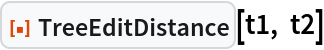Out=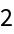### Applications (3)

Create two notebooks with similar content:

 In:=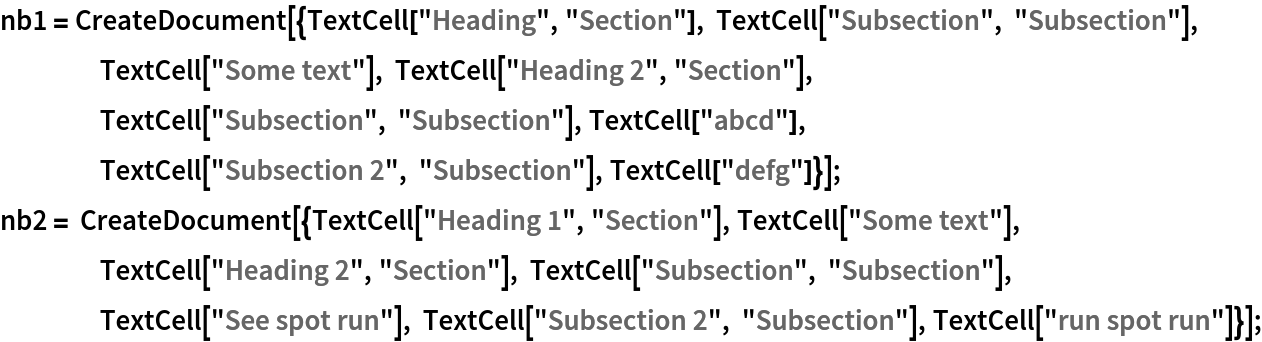Import the notebooks and convert to expression trees:

 In:=Out=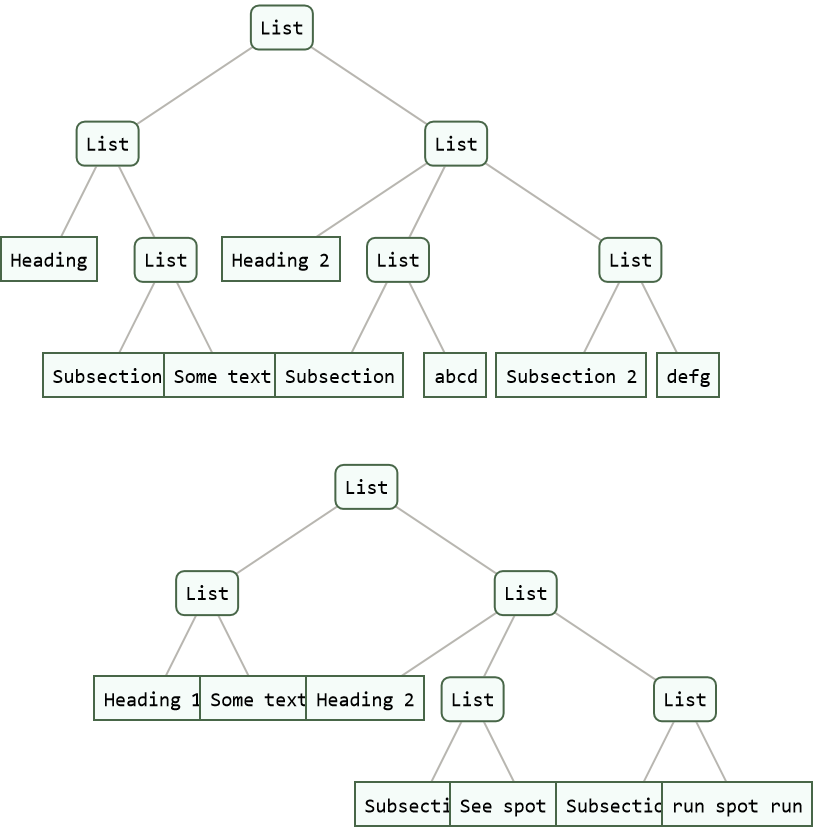Compute the edit distance between two expression trees:

 In:=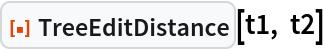Out=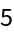Arnab Datta

## Version History

• 1.0.0 – 18 July 2022